Courses

# Flow Through Pipes Civil Engineering (CE) Notes | EduRev

## Topic wise GATE Past Year Papers for Civil Engineering

Created by: Gate Gurus

## Civil Engineering (CE) : Flow Through Pipes Civil Engineering (CE) Notes | EduRev

The document Flow Through Pipes Civil Engineering (CE) Notes | EduRev is a part of the Civil Engineering (CE) Course Topic wise GATE Past Year Papers for Civil Engineering.
All you need of Civil Engineering (CE) at this link: Civil Engineering (CE)

Question 1. Two identical pipes (i.e., having the same length, same diameter, and same roughness) are used to withdraw water from a reservoir. In the first case, they are attached in series and discharge freely into the atmosphere. In the second case, they are attached in parallel and also discharge freely into the atmosphere. Neglecting all minor losses, and assuming that the friction factor is same in both the cases, the ratio of the discharge in the parallel arrangement to that in the series arrangement (round off to 2 decimal places) is _______     [2019 : 2 Marks, Set-II]
Solution:
Given: Two identical pipes of same length (L), diameter (D) and roughness (ks).
1st case (Series)
Assume height of reservoir = H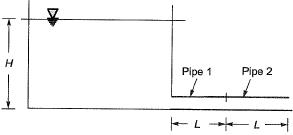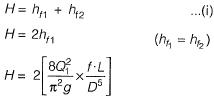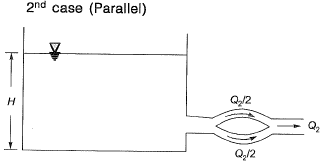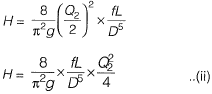By eq. (i) and (ii)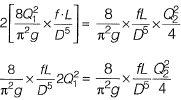=2.83 (round off to 2 decimal place)

Question 2. Two water reservoirs are connected by a siphon (running full) of total length 5000 m and diameter of 0.10 m, as shown below (figure not drawn to scale).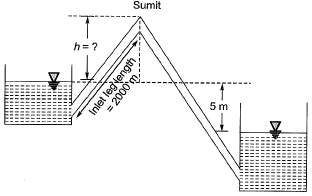The inlet leg length of the siphon to its summit is 2000 m. The difference in the water surface levels of the two reservoirs is 5 m. Assume the permissible minimum absolute pressure at the summit of siphon to be 2.5 m of water when running full. Given: friction factor, f = 0.02 throughout, atmospheric pressure — 10.3 m of water and acceleration due to gravity g = 9.81 m/s2. Considering only major loss using Darcy-Weisback equation, the maximum height of the summit of siphon from the water level of upper reservoir, h (in m, round off to 1 decimal p lace) is_______ .    [2019 : 2 Marks, Set-I]
Solution: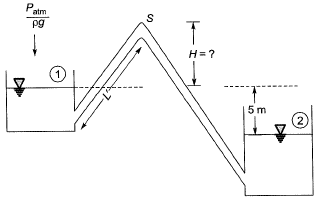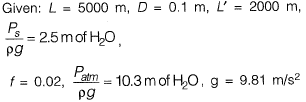Consider only major losses
Apply energy equation between (1) and (2)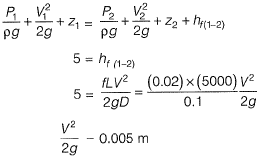Apply energy equation between (1) and (S)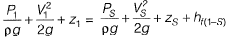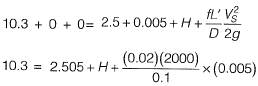10.3 = 2.505 + H + 2
H = 5.795
H = 5.8 m (upto 1 decimal place)

Question 3. Water is pumped at a steady uniform flow rate of 0.01 m3/s through a horizontal smooth circular pipe of 100 mm diameter. Given that the Reynolds number is 800 and g is 9.81 m/s2, the head loss (in meters, up to one decimal place) per km length due to friction would b e _________ .     [2017 ; 2 Marks. Set-II]
Solution: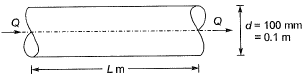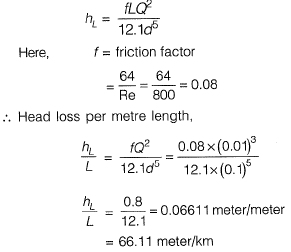Question 4. A triangular pipe network is shown in the figure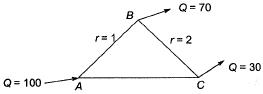The head loss in each pipe is given by hf = rQ1.8, with the variables expressed in a consistent set of units. The value of r for the pipe AB is 1 and for the pipe BC is 2. If the discharge supplied at the point A (i.e., 100) is equally divided between the pipes AB and AC, the value of r (up to 2 decimal places) for the pipe AC should be______    [2017 : 1 Mark, Set-I]
Solution:
Given hf = r . Q1.8
Because of the given condition of equal discharge distribution in pipe AB & AC, the discharge in AB and AC will be 50 and 50. Now satisfy continuity at point Band C, discharge from C to B will be 20.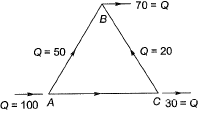For close Loop ABCA,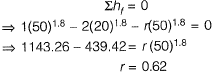Question 5. A pipe of 0.7 m diameter has a length of 6 km and connects two reservoirs A and B. The water level in reservoir A is at an elevation 30 m above the water level in reservoir B. Halfway along the pipe line, there is a branch through which water can be supplied to a third reservoir C. The friction factor of the pipe is 0.024. The quantity of water discharged into reservoir C is 0.15 m3/s. Considering the acceleration due to gravity as 9.81 m/s2 and neglecting minor losses, the discharge (in m3/s) into the reservoir B is [2015 : 2 Marks, Set-II]A pipe of 0.7 m diameter has a length of 6 km and connects two reservoirs A and B. The water level in reservoir A is at an elevation 30 m above the water level in reservoir B. Halfway along the pipe line, there is a branch through which water can be supplied to a third reservoir C. The friction factor of the pipe is 0.024. The quantity of water discharged into reservoir C is 0.15 m3/s. Considering the acceleration due to gravity as 9.81 m/s2 and neglecting minor losses, the discharge (in m3/s) into the reservoir B is______.     [2015 : 2 Marks, Set-II]
Solution: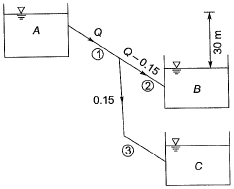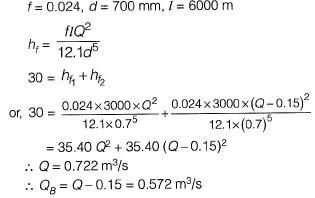Question 6. Two reservoirs are connected through a 930 m long, 0.3 m diameter pipe, which has a gate valve. The pipe entrance is sharp (loss coefficient = 0.5) and the valve is half-open (loss coefficient = 5.5). The head difference between the two reservoirs is 20 m. Assume the friction factor for the pipe as 0.03 and g = 10 m/s2. The discharge in the pipe accounting for all minor and major losses i s _____ m3/s.    [2015 : 2 Marks, Set-I]
Solution: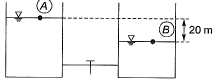Given:
f = 0.03, L = 930 m, D = 0.3 m , g = 10m/s2
Apply energy equation between A and B,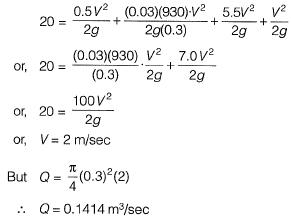Question 7. For steady incompressible flow through a closed- conduit of uniform cross-section, the direction of flow will always be     [2015 : 1 Mark, Set-I]
(a) from higher to lower elevation
(b) from higher to lower pressure
(c) from higher to lower velocity
(d) from higher to lower piezometric head
(d)
Solution:
In closed uniform conduit, velocity head remains constant, thus flow will be from higher to lower Piezometric head.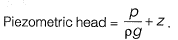Question 8. A straight 100 m long raw water gravity main is to carry water from an intake structure to the jack well of a water treatment plant. The required flow through this water main is 0.21 m3/s. Allowable velocity through the main is 0.75 m/s. Assume f = 0.01, g = 9.81 m/s2. The minimum gradient (in cm/100 m length) to be given to this gravity main so that the required amount of water flows without any difficulty is __________ .     [2014 : 2 Marks, Set-I]
Solution: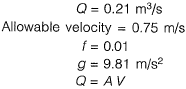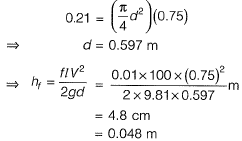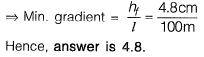Question 9. An incompressible fluid is flowing at a steady rate in a horizontal pipe. From a section, the pipe divides into two horizontal parallel pipes of diameters d1 and d2 (where d1 = 4d2) that run for a distance of L each and then again join back to a pipe of the original size. For both the parallel pipes, assume the head loss due to friction only and the Darcy-Weisbach friction factor to be the same. The velocity ratio between the bigger and the smaller branched pipes is ____ .     [2014 : 2 Marks, Set-I]
Solution: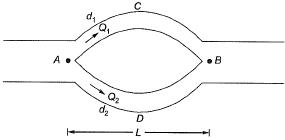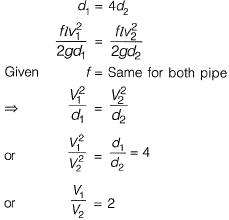Note: In this problem, Hagen-Poiseuille equation can't be applied because in the problem laminar flow is not mentioned.

Question 10. A 2 km pipe of 0.2 m diameter connects two reservoirs. The difference between the water levels in the reservoir is 8 m. The Darcy Weisbach friction factor of the pipe is 0.04. Accounting for frictional entry and exit losses. The velocity in the pipe in (m/sec) is    [2013 : 2 Marks]
(a) 0.63
(b) 0.35
(c) 2.52
(d) 1.25
(a)
Solution:
Frictional loss,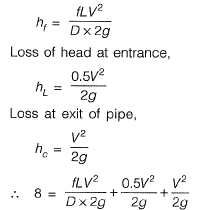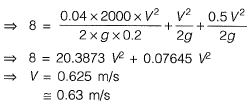Question 11.  The circular water pipes shown in the sketch are flowing full. The velocity of flow (in m/s) in the branch pipe “R” is    [2012 : 1 Mark]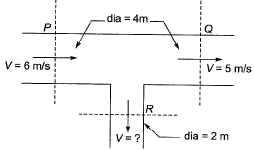(a) 3
(b) 4
(c) 5
(d) 6
(b)
Solution: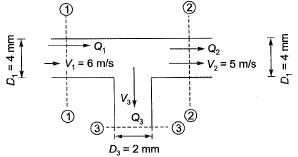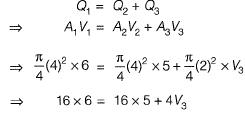=> 16 = 4 V3
=> V3 = 4 m/s

Question 12.  A single pipe of length 1500 m and diameter 60 cm connects two reservoirs having a difference of 20 m in their water levels. The pipe is to be replaced by two pipes of the same length and equal diameter 'd to convey 25% more discharge under the same head loss. If the friction factor is assumed to be the same for all the pipes, the value of ‘d ’ is approximately equal to which of the following options?    [2011 : 2 Marks]
(a) 37.5 cm
(b) 40.0 cm
(c) 45.0 cm
(d) 50.0 cm
(d)
Solution: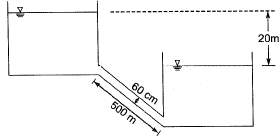For single pipe,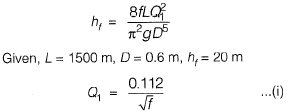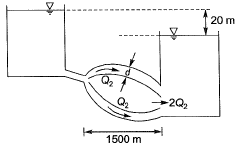In case of double pipe discharge is increased by 25% than that of previous case hence,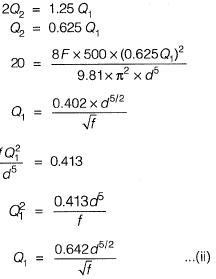From eq. (i) and (ii), we get,
d = 49.7 cm = 50 cm

Offer running on EduRev: Apply code STAYHOME200 to get INR 200 off on our premium plan EduRev Infinity!

,

,

,

,

,

,

,

,

,

,

,

,

,

,

,

,

,

,

,

,

,

;